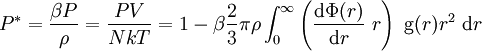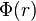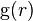# Difference between revisions of "Pressure equation"$P^*=\frac{\beta P}{\rho}= \frac{PV}{NkT} = 1 - \beta \frac{2}{3} \pi \rho \int_0^{\infty} \left( \frac{{\rm d}\Phi(r)} {{\rm d}r}~r \right)~{\rm g}(r)r^2~{\rm d}r$
where$\Phi(r)$ is a central potential and${\rm g}(r)$ is the pair distribution function.